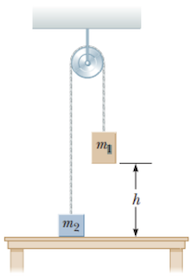# Problem: Two objects are connected by a light string passing over a light, frictionless pulley as shown in the figure. The object of mass m1 = 5.00 kg is released from rest at a height h = 4.00 m above the table. Using the isolated system model,(a) determine the speed of the object of mass m2 = 3.00 kg just as the 5.00-kg object hits the table(b) find the maximum height above the table to which the 3.00-kg object rises

###### FREE Expert Solution
91% (291 ratings)
###### Problem Details

Two objects are connected by a light string passing over a light, frictionless pulley as shown in the figure. The object of mass m1 = 5.00 kg is released from rest at a height h = 4.00 m above the table. Using the isolated system model,
(a) determine the speed of the object of mass m2 = 3.00 kg just as the 5.00-kg object hits the table
(b) find the maximum height above the table to which the 3.00-kg object risesFrequently Asked Questions

What scientific concept do you need to know in order to solve this problem?

Our tutors have indicated that to solve this problem you will need to apply the Energy in Connected Objects (Systems) concept. You can view video lessons to learn Energy in Connected Objects (Systems). Or if you need more Energy in Connected Objects (Systems) practice, you can also practice Energy in Connected Objects (Systems) practice problems.

How long does this problem take to solve?

Our expert Physics tutor, Julia took 4 minutes and 30 seconds to solve this problem. You can follow their steps in the video explanation above.

What professor is this problem relevant for?

Based on our data, we think this problem is relevant for Professor Markert's class at TEXAS.

What textbook is this problem found in?

Our data indicates that this problem or a close variation was asked in Physics for Scientists and Engineers - Serway Calc 9th Edition. You can also practice Physics for Scientists and Engineers - Serway Calc 9th Edition practice problems.